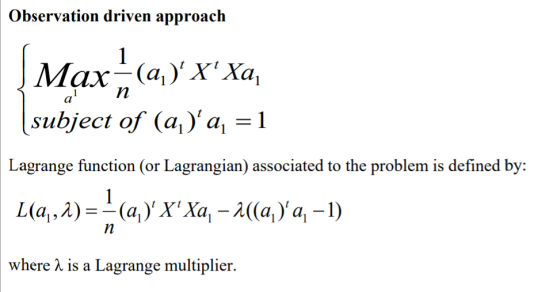# Principal Component Analysis - a short explanation

By Karina | Technophilia | 3 Mar 2021

The principal component analysis is a procedure that uses an orthogonal transformation to convert a set of data that has correlated variables into a set of uncorrelated variables called principal components. This is used to get rid of redundant information. An example is transforming the information from an Excel file into a table that still contains the important data.

Create a simple project in python, add the PCS.py class. After creating a folder in the python environment, in the Project tool window click  File|New, python file and name it PCS.py. In the constructor of the class add the matrix as a parameter. Please, don't forget to import NumPy, pandas, and PCS packets into your project. as shown in the last code part.

``````def __init__(self,X):

self.X=X``````

Before we go further I think that it is my duty to explain the theoretical and mathematical part. We want to calculate the covariance matrix. I chose the Observation driven approach.  The Lagrangian approach is written in the picture below.Therefore a1 is an eigenvector of the matrix 1/n(Xtransposed*X), n is the number of rows.  An eigenvector is any vector that only gets scaled.

Next, we have to provide the dimension or axis, averages on columns.

``````avgVar=np.mean(self.X,axis=0)

stdDevVar=np.std(self.X,axis=0)

self.Xstd=(self.X-avgVar)/stdDevVar``````

If we have the standardized matrix X noted as Xstd then we can use the covariance matrix like the one for which we compute the eigenvalues and the eigenvectors.

``````self.R=np.cov(self.Xstd,rowvar=False)

eigenVal,eigenVect=np.linalg.eigh(self.R)``````

After this, we will sort the eigenvalues and the eigenvectors in descending order.

``````kReverse=[k for k in reversed(np.argsort(eigenVal))]

self.alpha=eigenVal[kReverse]

self.a=eigenVect[kReverse]

self.C=Xstd @ self.a``````

We want to multiply the standardized matrix with the matrix of multipliers, eigenvectors. This self. C is the principal component.  The @ operator is used for multiplying two matrices. That is equivalent to using matmul() method which overloads @. If we would use matmult() we would have to write like this self.C = np.matmul(self.Xstd, self.a).

I wrote the functions for returning the values at the end of the class.

``````def getEigenValues(self):

return self.alpha

def getEigenVectors(self):

return self.a

def getPrincipalComponents(self):

return self.C``````

We will also need another file worksheet.py where we are using functions and variables from the PCA.py class and where we are going to read our file 'Teritorial.csv'.

``````import numpy as np
import PCA as pca
import pandas as pd
import graphics as grp

# what happens if we need random values in the interval [a, b], for any given a and b
def random(a=None, b=None, size=None) :         # [a, b]
return a + np.random.rand(size) * (b - a)

vector = random(1, 2, 35)
print(type(vector), vector)

X = np.ndarray(shape=(7, 5), dtype=float, buffer=vector, order='C')
print(X)

table = pd.read_csv('Teritorial.csv', sep=',', index_col=0) # we have the label of the rows on the first column
print(table)

obsName = table.index[:]
varName = table.columns[1:]

X = table.iloc[:, 1:].values
print(X)
print(obsName)
print(varName)

n = X.shape
m = X.shape
print('No of observations: ', n)
print('No of variables: ', m)

pcaModel = pca.PCA(X, reg=True)
# print(pcaModel.getEigenValues())``````

You might not need some lines of code printed in the worksheet.py, we will use them in the next chapter when I will talk more about the Covariance matrix and about graphics. I tried to cover both the teorethical and mathematical part and the code too. If you have any suggestions for my future explanations I will be more than happy to receive them.Karina

Some group of bits smashed into a soul that floats into this digital UniverseTechnophilia